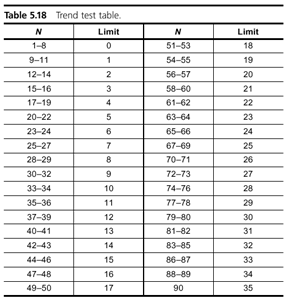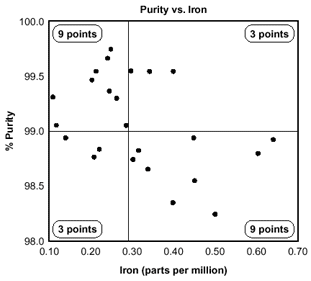Please note: We are currently experiencing high call volumes as we onboard our valued customers to our new system. We appreciate your patience and understanding.
| Cart Total:

# What is a Scatter Diagram?

Quality Glossary Definition: Scatter diagram

Also called: scatter plot, X-Y graph

The scatter diagram graphs pairs of numerical data, with one variable on each axis, to look for a relationship between them. If the variables are correlated, the points will fall along a line or curve. The better the correlation, the tighter the points will hug the line. This cause analysis tool is considered one of the seven basic quality tools.

## When to Use a Scatter Diagram

• When you have paired numerical data
• When your dependent variable may have multiple values for each value of your independent variable
• When trying to determine whether the two variables are related, such as:
• When trying to identify potential root causes of problems
• After brainstorming causes and effects using a fishbone diagram to determine objectively whether a particular cause and effect are related
• When determining whether two effects that appear to be related both occur with the same cause
• When testing for autocorrelation before constructing a control chart

## Scatter Diagram Procedure

1. Collect pairs of data where a relationship is suspected.
2. Draw a graph with the independent variable on the horizontal axis and the dependent variable on the vertical axis. For each pair of data, put a dot or a symbol where the x-axis value intersects the y-axis value. (If two dots fall together, put them side by side, touching, so that you can see both.)
3. Look at the pattern of points to see if a relationship is obvious. If the data clearly form a line or a curve, you may stop because variables are correlated. You may wish to use regression or correlation analysis now. Otherwise, complete steps 4 through 7.
4. Divide points on the graph into four quadrants. If there are X points on the graph:
• Count X/2 points from top to bottom and draw a horizontal line.
• Count X/2 points from left to right and draw a vertical line.
• If number of points is odd, draw the line through the middle point.
5. Count the points in each quadrant. Do not count points on a line.
6. Add the diagonally opposite quadrants. Find the smaller sum and the total of points in all quadrants.
A = points in upper left + points in lower right
B = points in upper right + points in lower left
Q = the smaller of A and B
N = A + B
7. Look up the limit for N on the trend test table.
• If Q is less than the limit, the two variables are related.
• If Q is greater than or equal to the limit, the pattern could have occurred from random chance.## Scatter Diagram Example

The ZZ-400 manufacturing team suspects a relationship between product purity (percent purity) and the amount of iron (measured in parts per million or ppm). Purity and iron are plotted against each other as a scatter diagram, as shown in the figure below.

There are 24 data points. Median lines are drawn so that 12 points fall on each side for both percent purity and ppm iron.

To test for a relationship, they calculate:
A = points in upper left + points in lower right = 9 + 9 = 18
B = points in upper right + points in lower left = 3 + 3 = 6
Q = the smaller of A and B = the smaller of 18 and 6 = 6
N = A + B = 18 + 6 = 24

Then they look up the limit for N on the trend test table. For N = 24, the limit is 6.
Q is equal to the limit. Therefore, the pattern could have occurred from random chance, and no relationship is demonstrated.Scatter Diagram Example

Below are some examples of situations in which might you use a scatter diagram:

• Variable A is the temperature of a reaction after 15 minutes. Variable B measures the color of the product. You suspect higher temperature makes the product darker. Plot temperature and color on a scatter diagram.
• Variable A is the number of employees trained on new software, and variable B is the number of calls to the computer help line. You suspect that more training reduces the number of calls. Plot number of people trained versus number of calls.
• To test for autocorrelation of a measurement being monitored on a control chart, plot this pair of variables: Variable A is the measurement at a given time. Variable B is the same measurement, but at the previous time. If the scatter diagram shows correlation, do another diagram where variable B is the measurement two times previously. Keep increasing the separation between the two times until the scatter diagram shows no correlation.

## Scatter Diagram Considerations

• Even if the scatter diagram shows a relationship, do not assume that one variable caused the other. Both may be influenced by a third variable.
• When the data are plotted, the more the diagram resembles a straight line, the stronger the relationship.
• If a line is not clear, statistics (N and Q) determine whether there is reasonable certainty that a relationship exists. If the statistics say that no relationship exists, the pattern could have occurred by random chance.
• If the scatter diagram shows no relationship between the variables, consider whether the data might be stratified.
• If the diagram shows no relationship, consider whether the independent (x-axis) variable has been varied widely. Sometimes a relationship is not apparent because the data do not cover a wide enough range.

## Scatter Diagram Resources

You can also search articles, case studies, and publications for scatter diagram resources.

### Books

The Quality Toolbox

### Articles

Pitch Perfect (Lean & Six Sigma Review) Learning the ins and outs of capability analysis by examining Pittsburgh Pirates starting pitcher Jameson Taillon’s performance using scatter diagrams.

Adapted from The Quality Toolbox, ASQ Quality Press.